# Lesson Notes By Weeks and Term - Primary 3

Division

TERM: 2nd Term

WEEK: 10

CLASS: Primary 3

AGE: 8 years

DURATION: 5 periods of 40 minutes each

DATE:

SUBJECT: Mathematics

TOPIC: Division

SPECIFIC OBJECTIVES: At the end of the lesson, the pupils should be able to

1. Recall multiplication facts for 10 times tables with answers up to 100.
2. Solve number problems in context and explain own solution to problems involving multiplication with answers up to 75, using appropriate symbols x, =, â¡.
3. Multiply 2, 3, 4, 5 to a total of 75, 100, 100 and 100 respectively
4. Divide numbers to 75 by 2, using appropriate symbols ÷, =, â¡,Divide numbers to 99 by 3, using appropriate symbols ÷, =, â¡,Divide numbers to 99 by 5, using appropriate symbols ÷, =, â¡ and Divide numbers to 99 by 4, using appropriate symbols ÷, =, â¡.

INSTRUCTIONAL TECHNIQUES: Explanation, question and answer, demonstration, practical, assessments

INSTRUCTIONAL MATERIALS: Multiplication table grid, counters (optional/remediation), videos from source-

PERIOD 1: Multiplication and division

PRESENTATION

TEACHER’S ACTIVITY

PUPIL’S ACTIVITY

STEP 1

MENTAL MATHS

The teacher begins the lesson with some mental calculations

Calculate

1. 5 x 10 =

2. 2 x 10 =

3. 7 x 10 =

4. 1 x 10 =

5. 4 x 10 =

6. 3 x 10 =

7. 10 x 10 =

8. 0 x 10 =

9. 6 x 10 =

10. 8 x 10 =

Pupils respond and participate

STEP 2

CONCEPT

DEVELOPMENT

The teacher

Revises using arrays.

• Draws an array on the board with 2 columns and 10 rows.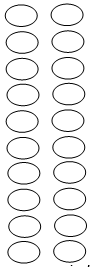-How many circles are there in each row? (2)

- How many circles are there altogether? Count: (2, 4, 6, 8, 10, 12, 14, 16, 18 and 20)

Let us write this as an addition number sentence: (2 + 2 + 2 + 2 + 2 + 2 + 2 + 2 + 2 + 2 = 20)

Let us write this as a multiplication number sentence: (2 x 10 = 20 or 10 x 2 = 20)

The inverse of multiplication is division.

-What would a division number sentence using this array look like?

(20 ÷ 2 = 10, 20 ÷ 10 = 2)

CLASS ACTIVITY

The multiplication table. Grade 3s can start to drill basic number facts – this

is the table with all of the basic multiples – highlighting the multiples of 2.

• The focus is on the language, which allows a

mental image for grouping.

(E.g. one 2 is two, two 2s are four, etc.)

 1 2 3 4 5 6 7 8 9 10 1 1 2 3 4 5 6 7 8 9 10 2 2 4 6 8 10 12 14 16 18 20 3 3 6 9 12 15 18 21 24 27 30 4 4 8 12 16 20 24 28 32 36 40 5 5 10 15 20 25 30 35 40 45 50 6 6 12 18 24 30 36 42 48 54 60 7 7 14 21 28 35 42 49 56 63 70 8 8 16 24 32 40 48 56 64 72 80 9 9 18 27 36 45 54 63 72 81 90 10 10 20 30 40 50 60 70 80 90 100

ACTIVITY II

Word problems involving repeated sets.

A vegetable garden has 4 rows of plants. Each row has 2 plants. How many plants are there in the garden?

Let us write it as an addition number sentence: (2 + 2 + 2 + 2 = â¡)

We can say there are 4 rows with 2 plants in each row. Draw a picture if necessary.

Let us write it as a multiplication number sentence: (4 x 2 = â¡)

• The teacher asks learners to make up other stories that lead to multiplication – where repeated sets are involved.

E.g. A car can take 5 passengers. How many passengers can 2 cars take? (5 x 2 = 10)

ACTIVITY III

Division – grouping.

If I put 62 shoes into pairs, how many pairs of shoes will I have?

• Let us write this as a number sentence: 62 ÷ 2 = 31

• Do the same by making stories for putting different numbers of items into pairs:

−− 26 (13)

−− 8 (19)

−− 44 (22)

−− 48 (24).

Pupils pay attention and participate

STEP 3

CLASS-WORK

1. Complete these flow diagrams2. The manager has to order tyres for 35 bicycles.

If each bicycle needs two tyres, how many tyres must the manager order?

3. If two learners fit into a car how many cars will take 24 learners?

Pupils attempt their class work

STEP 4

HOME-WORK

1. In the shop there are 23 pairs of shoes. How many single shoes are there?

______

2. A teacher gives out 2 books to each child in her class. She gives out 68 books.

How many children are in her class? ______

3. Calculate:

a) 22 ÷ 2 = __

b) 28 ÷ 2 = __

c) 20 ÷ 2 = __

d) 32 ÷ 2 = __

Pupils attempt their class work

STEP 5

SUMMARY

The teacher summarizes by reminding the pupils how to multiply and divide

She marks their class works, makes corrections where necessary and commends them positively

PERIOD 2 : Multiplication and division

PRESENTATION

TEACHER’S ACTIVITY

PUPIL’S ACTIVITY

STEP 1

MENTAL MATHS

The teacher begins the lesson with some mental calculations

Calculate

1. 3 x 10 =

2. 9 x 10 =

3. 0 x 10 =

4. 8 x 10 =

5. 4 x 10 =

6. 2 x 10 =

7. 10 x 10 =

8. 5 x 10 =

9. 7 x 10 =

10. 6 x 10 =

Pupils respond and participate

STEP 2

CONCEPT

DEVELOPMENT

The teacher

Revise using arrays.

• Draw the array on the board.

How many circles are there in each row? (3)

How many circles are there altogether? Count: (3, 6, 9, 12, 15, 18, 21, 24, 27, 30)

(3 + 3 + 3 + 3 + 3 + 3 + 3 + 3 + 3 + 3 = 30)

• Ask learners to write this using a multiplication number sentence: (3 x 10 = 30 or 10 x 3 = 30)

• Ask learners to write a division number sentence: (30 ÷ 3 = 10 or 30 ÷ 10 = 3)CLASS ACTIVITY

The multiplication table. In this lesson you work with the table with

all of the basic multiples – highlighting the multiples of 3.

• The focus is on the language, which allows a mental image for

grouping.

(E.g. one 3 is three, two 3s are six, etc.)

 1 2 3 4 5 6 7 8 9 10 1 1 2 3 4 5 6 7 8 9 10 2 2 4 6 8 10 12 14 16 18 20 3 3 6 9 12 15 18 21 24 27 30 4 4 8 12 16 20 24 28 32 36 40 5 5 10 15 20 25 30 35 40 45 50 6 6 12 18 24 30 36 42 48 54 60 7 7 14 21 28 35 42 49 56 63 70 8 8 16 24 32 40 48 56 64 72 80 9 9 18 27 36 45 54 63 72 81 90 10 10 20 30 40 50 60 70 80 90 100

ACTIVITY II

Word problems involving multiplicative comparison.

I want to make 4 cakes and for every cake I need 3 cups of flour. How many cups of flour do I need?

(4 x 3 = 12)

• You can draw this as a rectangular array (three by four).

• Let us write it as an addition number sentence: (3 + 3 + 3 + 3 = 12)

• Let us write it as a multiplication number sentence: (4 x 3 = â¡)

• Ask learners to make up other stories that lead to multiplication – where multiplicative comparison involved.

E.g. There are 3 times as many blue counters as there are white counters in the box. If there are 7 white

counters in the box, how many blue counters are there? (7 x 3 = 10)

ACTIVITY III

Division – sharing.

• If I have 42 biscuits and I share them between 3 learners, how many biscuits will each learner get?

Let us write this as a number sentence: (42 ÷ 3 = 14)

Tell another story about the division number sentence. (Mum shares 42 buttons among 3 children. Each child

gets 14 buttons.)

• Do the same for 27, 39, 48, 54. If you share each of these numbers of biscuits between three learners, how many biscuits will they get each time? (9, 13, 16, 18)

Pupils pay attention and participate

STEP 3

CLASS-WORK

1. Complete these flow diagrams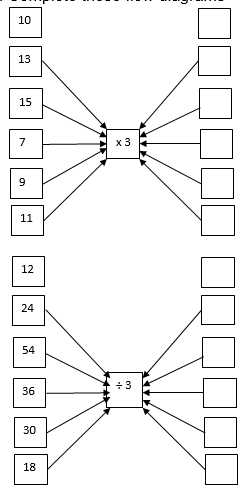2. The nursery school teacher has to order tyres for 9 tricycles. If each tricycle needs three tyres,

how many tyres must the nursery school teacher order?

a) 10 x 3 = 30

b) 12 ÷ 3 = 4

Pupils attempt their class work

STEP 4

HOME-WORK

Look at the array below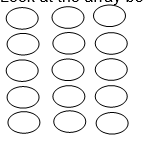1. Write an addition number sentence.

2. Write a multiplication number sentence.

3. Write a story about the multiplication number sentence.

4. Write a division number sentence.

5. Write a story about the division number sentence.

Pupils attempt their class work

STEP 5

SUMMARY

The teacher summarizes by reminding the pupils how to multiply and divide numbers in 3s

She marks their class works, makes corrections where necessary and commends them positively

PERIOD 3: Multiplication and division

PRESENTATION

TEACHER’S ACTIVITY

PUPIL’S ACTIVITY

STEP 1

MENTAL MATHS

The teacher begins the lesson with some mental calculations

Calculate

Double the following

1. 8

2. 6

3. 0

4. 50

5. 40

Half the following

6. 20

7. 80

8. 100

9. 0

10. 16

Pupils respond and participate

STEP 2

CONCEPT

DEVELOPMENT

The teacher

Revise using arrays.

• Draw the 4 x 5 array on the board.

• Let us count in 5s: 5, 10, 15, 20.

• Write this using an addition number sentence: 5 + 5 + 5 + 5 = 20.

• Write this using a multiplication number sentence: 4 x 5 = 20 or 5 x 4 = 20.

• Write this using a division number sentence: 2 ÷ 5 = 4 or 20 ÷ 4 = 5.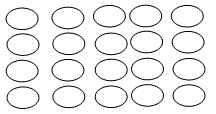CLASS ACTIVITY

The multiplication table.

Let learners use the multiplication board to build up their 5 times tables, e.g. one 5 is five, two 5s are ten, etc.

 1 2 3 4 5 6 7 8 9 10 1 1 2 3 4 5 6 7 8 9 10 2 2 4 6 8 10 12 14 16 18 20 3 3 6 9 12 15 18 21 24 27 30 4 4 8 12 16 20 24 28 32 36 40 5 5 10 15 20 25 30 35 40 45 50 6 6 12 18 24 30 36 42 48 54 60 7 7 14 21 28 35 42 49 56 63 70 8 8 16 24 32 40 48 56 64 72 80 9 9 18 27 36 45 54 63 72 81 90 10 10 20 30 40 50 60 70 80 90 100

ACTIVITY II

Word problems – multiplication involving repeated sets.

• My dad planted 5 fruit trees in a row. He planted 6 rows.

How many fruit trees did he plant? (30)

• Let us write it as an addition number sentence: 5 + 5 + 5 + 5 + 5 + 5 = â¡ (30)

• Let us write it as a multiplication number sentence: 6 x 5 = â¡ (30)

• Ask learners to make up other stories that lead to multiplication where repeated sets are involved.

E.g. A car can take 5 passengers. How many passengers can 3 cars take? (5 x 3 = 10)

ACTIVITY III

Word problems: division – grouping.

• You have 30 peaches and you put them into groups of 5.

How many groups would you make? (6)

• Let us write it as a division number sentence: 30 ÷ 5 = 6. There are 6 groups.

• Do the same with 40 peaches (8 groups), 50 peaches (10 groups), and 75 peaches (15 groups).

Pupils pay attention and participate

STEP 3

CLASS-WORK

1. Complete these flow diagrams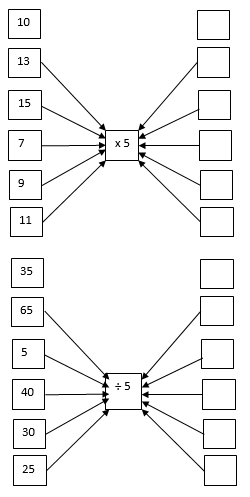2. If the baker uses 5 eggs each day, how long will 75 eggs last?

3. If the baker uses 5 eggs each day, how many eggs will he use in 13 days?

Pupils attempt their class work

STEP 4

HOME-WORK

1. Uncle Thomas keeps N5 coins in the ashtray of his car. He has eleven N5 coins.

How much money does he have in his ashtray?

2.a) Write an addition number sentence.

b) Write a subtraction number sentence.

c) Write a multiplication number sentence.

d) Write a division number sentence.

Pupils attempt their class work

STEP 5

SUMMARY

The teacher summarizes by reminding the pupils how to multiply and divide in 5s

She marks their class works, makes corrections where necessary and commends them positively

PERIOD 4: Multiplication and division

PRESENTATION

TEACHER’S ACTIVITY

PUPIL’S ACTIVITY

STEP 1

MENTAL MATHS

The teacher begins the lesson with some mental calculations

Calculate

1. 10 x 10 =

2. 8 x 10 =

3. 4 x 10 =

4. 6 x 10 =

5. 3 x 10 =

6. 100 ÷ 10 =

7. 80 ÷ 10 =

8. 40 ÷ 10 =

9. 60 ÷ 10 =

10. 30 ÷ 10 =

Pupils respond and participate

STEP 2

CONCEPT

DEVELOPMENT

The teacher

Revise using arrays.

• Draw an array with 4 circles and 10 rows on the board.

How many counters are in each row? (4)

Write this using an addition number sentence. (4 + 4 + 4 + 4 + 4 + 4 + 4 + 4 + 4 + 4 = 40)

What will a multiplication number sentence for this look like? (4 x 10 = 40 or 10 x 4 = 40)

The inverse of multiplication is division.

What would a division number sentence look like? (40 ÷ 4 = 10 or 40 ÷ 10 = 4)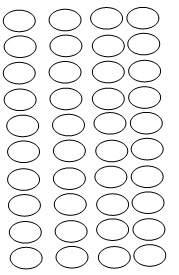CLASS ACTIVITY

The multiplication table. In this lesson you work with the table with all of the basic multiples – highlighting the multiples of 3.

• Give learners multiplication tables. Learners place fingers on the multiplication table to show one 4 is four, two 4s are eight, etc.

 1 2 3 4 5 6 7 8 9 10 1 1 2 3 4 5 6 7 8 9 10 2 2 4 6 8 10 12 14 16 18 20 3 3 6 9 12 15 18 21 24 27 30 4 4 8 12 16 20 24 28 32 36 40 5 5 10 15 20 25 30 35 40 45 50 6 6 12 18 24 30 36 42 48 54 60 7 7 14 21 28 35 42 49 56 63 70 8 8 16 24 32 40 48 56 64 72 80 9 9 18 27 36 45 54 63 72 81 90 10 10 20 30 40 50 60 70 80 90 100

ACTIVITY II

The multiplication table. In this lesson you work with the table with all of the basic multiples – highlighting the multiples of 3.

• Give learners multiplication tables. Learners place fingers on the multiplication table to show one 4 is four, two 4s are eight, etc.

ACTIVITY III

Word problems involving multiplicative comparison.

For every row in a vegetable garden there are 4 plants.

If there are 5 rows in the vegetable garden, how many plants are there?

• You can draw this using a rectangular array.

• Let us write it as an addition number sentence: 4 + 4 + 4 + 4 + 4 = â¡

• We can count: 4, 8, 12, 16, 20 plants

• Let us write it as a multiplication number sentence: 5 x 4 = â¡

Make up another story that leads to a multiplicative comparison of numbers.ACTIVITY III

Division – sharing.

If I have 68 cups and share them between four classes, how many cups will each class get?

• Write this as a number sentence: 68 ÷ 4 = 17

• Share items into 4 groups – try to make a story for each one with the learners.

• For example share 60 sweets between 4 classes (15), share 52 counters between 4 learners (13),

share 28 books between 4 boys (7).

Pupils pay attention and participate

STEP 3

CLASS-WORK

1. Complete these flow diagrams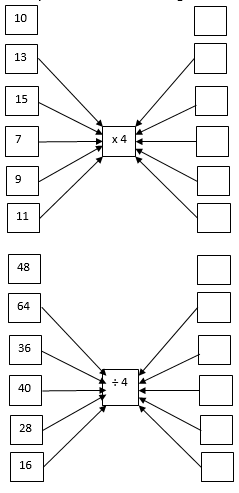2. Samuel has 68 sweets. He has four times as many sweets as Mohammed.

How many sweets does Mohammed have?

3. A vegetable garden has 4 rows of plants. Each row has 15 plants.

How many plants are there in the garden?

4. Write a story for:

a) 5 x 4 = 20

b) 24 ÷ 4 = 6

Pupils attempt their class work

STEP 4

HOME-WORK

Calculate and write a story for:

a) 17 x 4 = __ (68)

b) 36 ÷ 4= __ (9)

Pupils attempt their class work

STEP 5

SUMMARY

The teacher summarizes by reminding the pupils how to multiply and divide in 4s

She marks their class works, makes corrections where necessary and commends them positively

PERIOD 5: Weekly Test/consolidations

TEACHER’S ACTIVITY: The teacher revises all the concepts treated from period 1-4 and gives the pupils follow through exercises, quiz and tests. She marks the exercises, makes corrections and commends the pupils positively.

PUPIL’S ACTIVITY: The pupils work on the worksheets and exercises given by the teacher individually

CONSOLIDATION

1a. Draw and complete the following table

 x 1 2 3 4 5 6 7 8 9 10 3 6

b.

 ÷ 6 12 18 24 30 36 42 48 54 60 6 3

c.

 x 1 2 3 4 5 6 7 8 9 10 2 4

d.

 ÷ 10 20 30 40 50 60 70 80 90 100 5 10